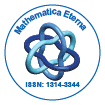# Mathematica EternaOpen Access

ISSN: 1314-3344

## Abstract

### Reduction of Higher Order Linear Ordinary Differential Equations into the Second Order and Integral Evaluation of Exact Solutions

Gunawan Nugroho, Ahmad Zaini and Purwadi A. Darwito

Higher order linear differential equations with arbitrary order and variable coefficients are reduced in this work. The method is based on the decomposition of their coefficients and the approach reduces the order until second order equation is produced. The method to find closedform solutions to the second order equation is then developed. The solution for the second order ODE is produced by rearranging its coefficients. Exact integral evaluation is also conducted to complete the solutions.

Top